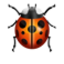# I got this message : Can't get the length of a `Hand` eventhough I'm not asked to provide the __len__ meth for hand cld

dice.py
```import random

class Die:
def __init__(self, sides=2):
if sides < 2:
raise ValueError("Can't have fewer than two sides")
self.sides = sides
self.value = random.randint(1, sides)

def __int__(self):
return self.value

return int(self) + other

return self + other

class D20(Die) :
def __init__(self) :
super().__init__(sides=20)
```
hands.py
```from dice import D20
class Hand(list):
@property
def total(self):
return sum(self)

def roll(self, number_of_dice) :
slots = []
for _ in range(number_of_dice) :
slots = []
slots.append(D20())
return slots
```Please report this to the Support staff and collect your "Exterminator"badge for being the first to find it.